### An example of get values from the iRSI indicator

Code: iRSI get value.mq5

Do not forget the rules: create the indicator handle ONCE in OnInit,

```//+------------------------------------------------------------------+
//| Expert initialization function                                   |
//+------------------------------------------------------------------+
int OnInit()
{
//--- create handle of the indicator iRSI
handle_iRSI=iRSI(Symbol(),Period(),Inp_RSI_ma_period,Inp_RSI_applied_price);
//--- if the handle is not created
if(handle_iRSI==INVALID_HANDLE)
{
//--- tell about the failure and output the error code
PrintFormat("Failed to create handle of the iRSI indicator for the symbol %s/%s, error code %d",
Symbol(),
EnumToString(Period()),
GetLastError());
//--- the indicator is stopped early
return(INIT_FAILED);
}
//---
return(INIT_SUCCEEDED);
}```

use CopyBuffer to get the data:

```//+------------------------------------------------------------------+
//| Expert tick function                                             |
//+------------------------------------------------------------------+
void OnTick()
{
//---
double rsi[];
ArraySetAsSeries(rsi,true);
int start_pos=0,count=2;
if(!iGetArray(handle_iRSI,0,start_pos,count,rsi))
return;
//---
string text="";
int limit=(count>2)?2:count;
for(int i=0; i<limit; i++)
{
text=text+
" bar #"+IntegerToString(i)+": "+
" rsi "+DoubleToString(rsi[i],2)+"\n";
}
Comment(text);
}```

Result: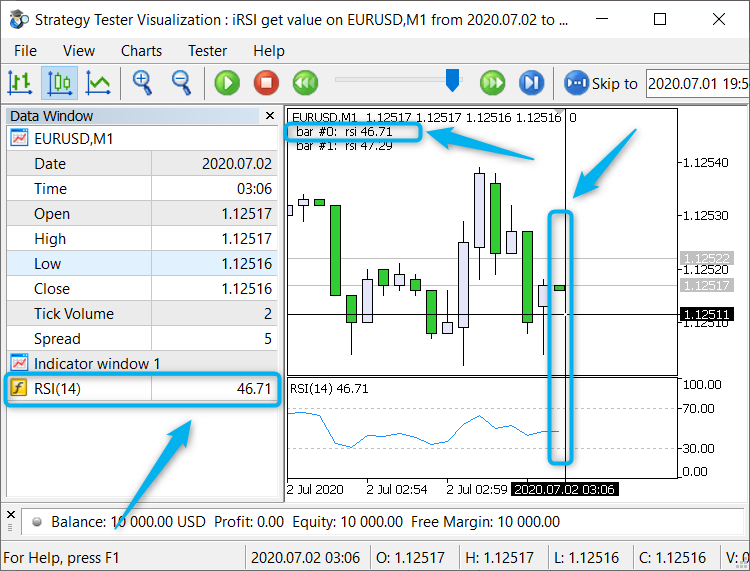Рис. 1. iRSI get value

Files:

### An example of get values from the iBands indicator

Code (Expert): iBands values on a chart.mq5

Do not forget the rules: create the indicator handle ONCE in OnInit,

```//+------------------------------------------------------------------+
//| Expert initialization function                                   |
//+------------------------------------------------------------------+
int OnInit()
{
//--- create handle of the indicator iBands
handle_iBands=iBands(Symbol(),Period(),Inp_Bands_bands_period,
Inp_Bands_bands_shift,Inp_Bands_deviation,Inp_Bands_applied_price);
//--- if the handle is not created
if(handle_iBands==INVALID_HANDLE)
{
//--- tell about the failure and output the error code
PrintFormat("Failed to create handle of the iBands indicator for the symbol %s/%s, error code %d",
Symbol(),
EnumToString(Period()),
GetLastError());
//--- the indicator is stopped early
return(INIT_FAILED);
}
//---
return(INIT_SUCCEEDED);
}```

use CopyBuffer to get the data:

```//+------------------------------------------------------------------+
//| Expert tick function                                             |
//+------------------------------------------------------------------+
void OnTick()
{
//---
string text="";
double upper[],lower[],middle[];
ArraySetAsSeries(upper,true);
ArraySetAsSeries(lower,true);
ArraySetAsSeries(middle,true);
int start_pos=0,count=3;
if(!iGetArray(handle_iBands,UPPER_BAND,start_pos,count,upper) ||
!iGetArray(handle_iBands,LOWER_BAND,start_pos,count,lower) ||
!iGetArray(handle_iBands,BASE_LINE,start_pos,count,middle))
{
return;
}
string text_upper="",text_lower="",text_middle="";
for(int i=count-1; i>=0; i--)
{
text_upper  = text_upper   + "Upper" + "["+IntegerToString(i) + "]" +" " + DoubleToString(upper[i],Digits()+1)  +" | ";
text_lower  = text_lower   + "Lower" + "["+IntegerToString(i) + "]" +" " + DoubleToString(lower[i],Digits()+1)  +" | ";
text_middle = text_middle  + "Middle"+ "["+IntegerToString(i) + "]" +" " + DoubleToString(middle[i],Digits()+1) +" | ";
}
Comment(text_upper+"\n"+text_lower+"\n"+text_middle);
}```

Result: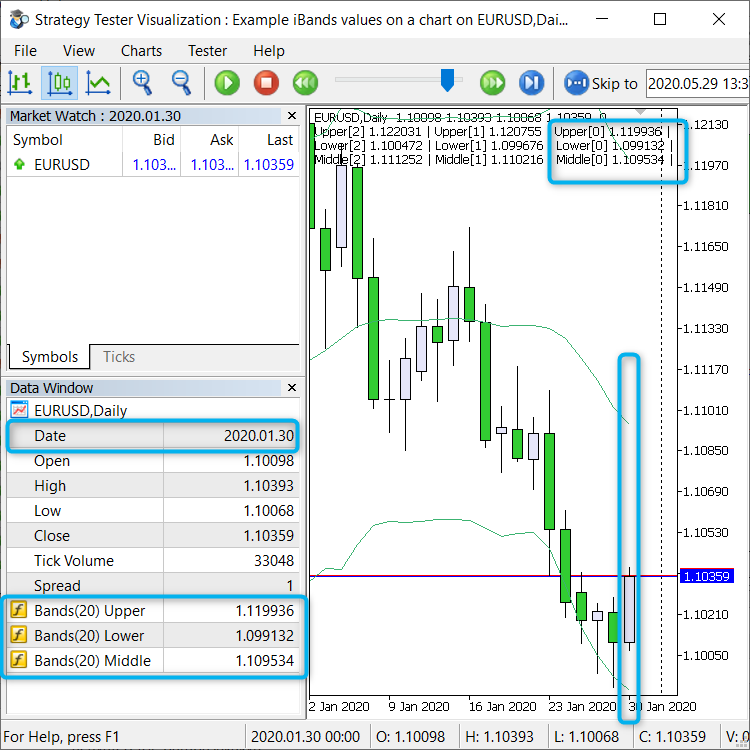Рис. 1.  iBands values on a chart

Files:

### Example of creating an indicator based on iATR

code: xSuperTrend.mq5

Step 1: In the MetaEditor, go to the folder with indicators (in my example, this is the "MyInd" folder). Next click the right mouse button: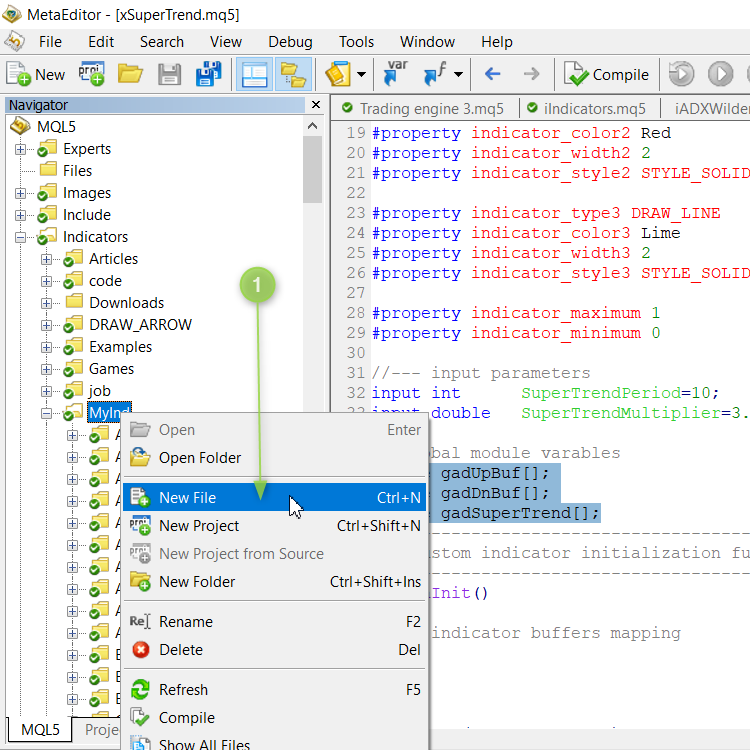Pic. 1. Step 1

Step 2: Choose the type of program: Custom Indicator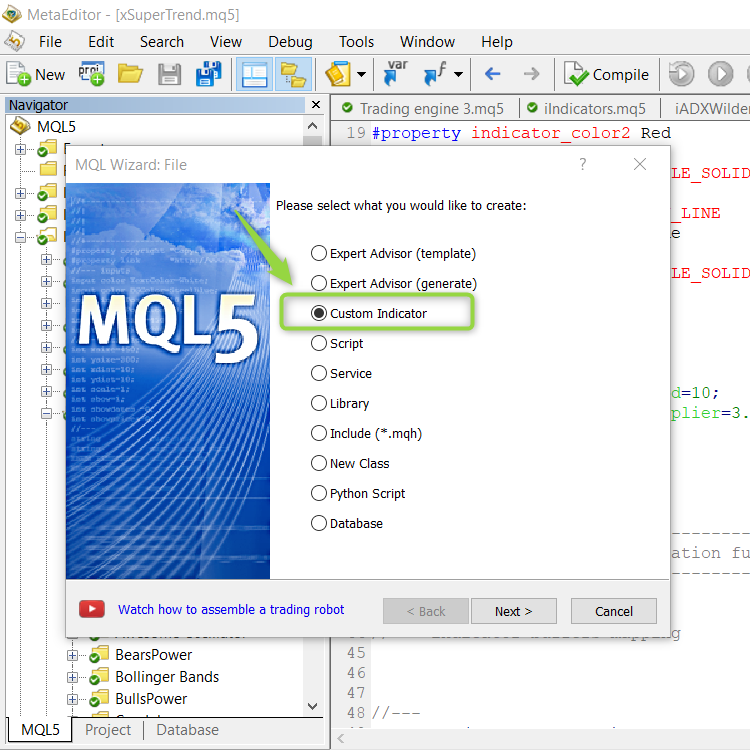Pic. 2. Step 2

Step 3: Enter the name of the indicator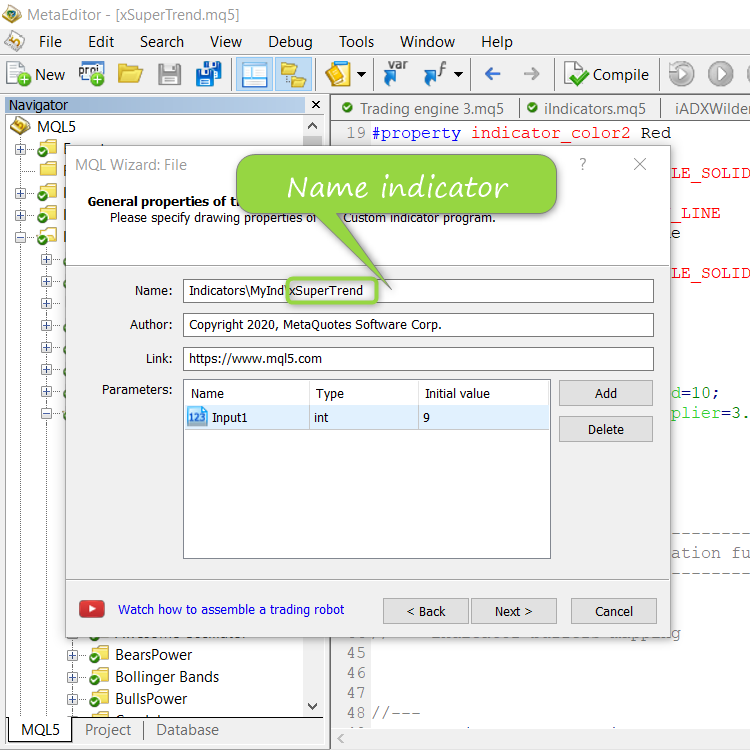Pic. 3. Step 3

Step 4. We connect the full form for OnCalculate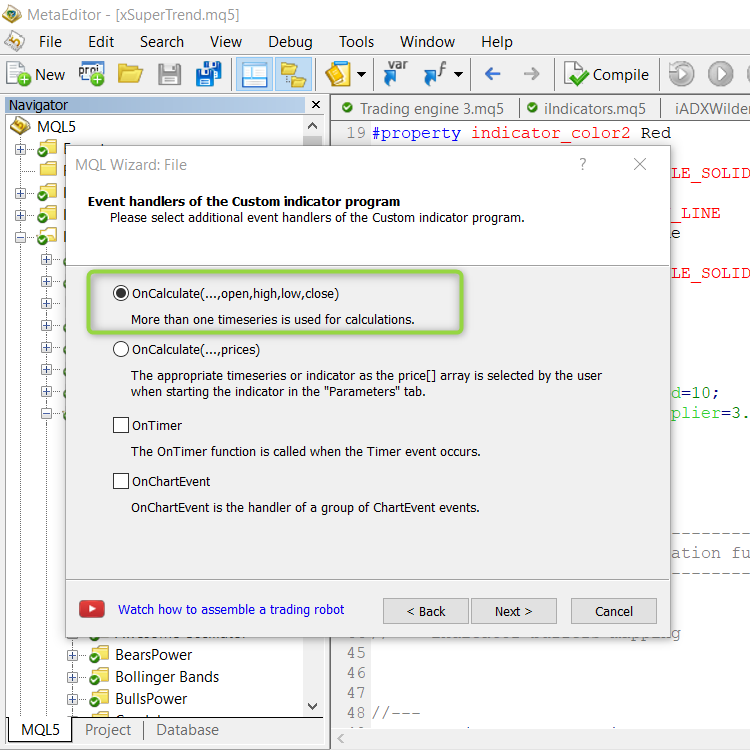Pic. 4. Step 4

Step 5. We add three graphical constructions to the indicator. We give them names and colors.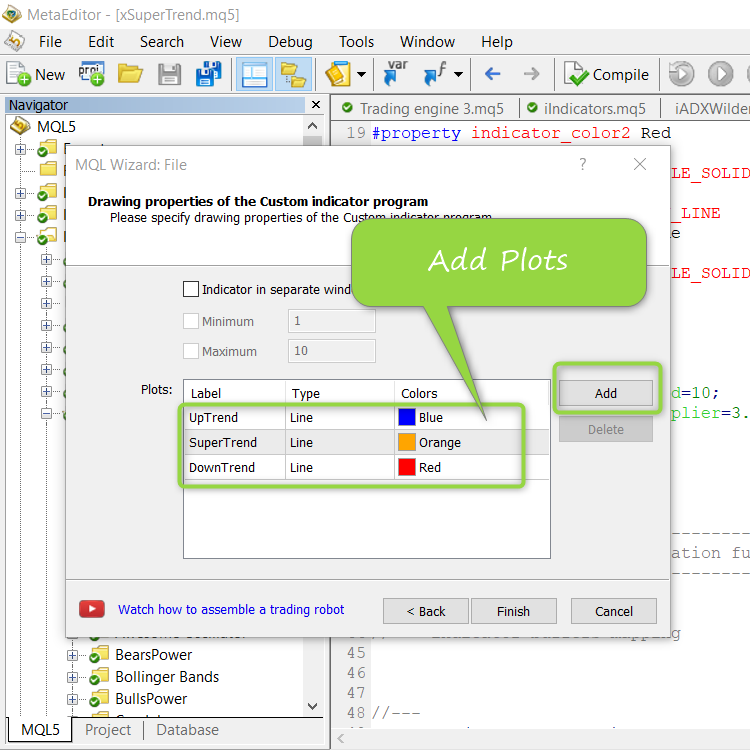Pic. 5. Step 5

As a result, we get a blank indicator. Denote the version with the number '1.000'

To be continued ...

Files:

### Example of creating an indicator based on iATR. Part 2.

code: xSuperTrend.mq5

Let's start filling our indicator: add input parameters, add the variable "handle_iATR" - the handle of the iATR indicator will be stored in it.

```#property indicator_width3  1
//--- input parameters
input int      Inp_ma_period           = 10;    // Super Trend: averaging period
input double   Inp_multiplier          = 3.0;   // Super Trend: multiplier
//--- indicator buffers
double   UpTrendBuffer[];
double   SuperTrendBuffer[];
double   DownTrendBuffer[];
//---
int      handle_iATR;                          // variable for storing the handle of the iATR indicator
//+------------------------------------------------------------------+
//| Custom indicator initialization function                         |
//+------------------------------------------------------------------+
int OnInit()```

Note: the variable 'handle_iATR' is declared at the global program level (in the "header" of the program).

Save the version number 1.001

To be continued ...

Files:

### Example of creating an indicator based on iATR. Part 3.

code: xSuperTrend.mq5

Works with help ( iATR )

Our indicator ('xSuperTrend') is equipped with iATR - which means we need one more buffer. Add a buffer.

It was:

```#property indicator_chart_window
#property indicator_buffers 3
#property indicator_plots   3

***
//--- indicator buffers
double   UpTrendBuffer[];
double   SuperTrendBuffer[];
double   DownTrendBuffer[];
//---
int      handle_iATR;                          // variable for storing the handle of the iATR indicator```

It became:

```#property indicator_chart_window
#property indicator_buffers 4
#property indicator_plots   3

***
//--- indicator buffers
double   UpTrendBuffer[];
double   SuperTrendBuffer[];
double   DownTrendBuffer[];
double   iATRBuffer[];
//---
int      handle_iATR;                          // variable for storing the handle of the iATR indicator```

We get the handle of the indicator (do not forget: the handle needs to be received ONE time in OnInit)

```//+------------------------------------------------------------------+
//| Custom indicator initialization function                         |
//+------------------------------------------------------------------+
int OnInit()
{
//--- indicator buffers mapping
SetIndexBuffer(0,UpTrendBuffer,INDICATOR_DATA);
SetIndexBuffer(1,SuperTrendBuffer,INDICATOR_DATA);
SetIndexBuffer(2,DownTrendBuffer,INDICATOR_DATA);
SetIndexBuffer(3,iATRBuffer,INDICATOR_CALCULATIONS);
//--- create handle of the indicator iATR
handle_iATR=iATR(Symbol(),Period(),Inp_ma_period);
//--- if the handle is not created
if(handle_iATR==INVALID_HANDLE)
{
//--- tell about the failure and output the error code
PrintFormat("Failed to create handle of the iATR indicator for the symbol %s/%s, error code %d",
Symbol(),
EnumToString(Period()),
GetLastError());
//--- the indicator is stopped early
return(INIT_FAILED);
}
//---
return(INIT_SUCCEEDED);
}```

Save the version number 1.002

To be continued ...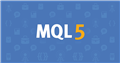Documentation on MQL5: Technical Indicators / iATR
• www.mql5.com
//|                                                    Demo_iATR.mq5 | //|                        Copyright 2011, MetaQuotes Software Corp. | //|                                              https://www.mql5.com | "The method of creation of the handle is set through the 'type' parameter (function type...
Files:

### Example of creating an indicator based on iATR. Part 4.

code: xSuperTrend.mq5

Works with help ( iATR ) - Copy paste :)

First, at the "top of the program", add a variable 'bars_calculated' - it stores the number of barors that the iATR indicator has counted.

```int      handle_iATR;                           // variable for storing the handle of the iATR indicator
int      bars_calculated=0;                     // we will keep the number of values in the Average True Range indicator
//+------------------------------------------------------------------+
//| Custom indicator initialization function                         |
//+------------------------------------------------------------------+
int OnInit()```

We almost completely insert the code from the help (In the inserted code, replace 'handle' with 'handle_iATR')

```//+------------------------------------------------------------------+
//| Custom indicator iteration function                              |
//+------------------------------------------------------------------+
int OnCalculate(const int rates_total,
const int prev_calculated,
const datetime &time[],
const double &open[],
const double &high[],
const double &low[],
const double &close[],
const long &tick_volume[],
const long &volume[],
{
//---
int values_to_copy;
//--- determine the number of values calculated in the indicator
int calculated=BarsCalculated(handle_iATR);
if(calculated<=0)
{
PrintFormat("BarsCalculated() returned %d, error code %d",calculated,GetLastError());
return(0);
}
//--- if it is the first start of calculation of the indicator or if the number of values in the iATR indicator changed
//---or if it is necessary to calculated the indicator for two or more bars (it means something has changed in the price history)
if(prev_calculated==0 || calculated!=bars_calculated || rates_total>prev_calculated+1)
{
//--- if the iATRBuffer array is greater than the number of values in the iATR indicator for symbol/period, then we don't copy everything
//--- otherwise, we copy less than the size of indicator buffers
if(calculated>rates_total)
values_to_copy=rates_total;
else
values_to_copy=calculated;
}
else
{
//--- it means that it's not the first time of the indicator calculation, and since the last call of OnCalculate()
//--- for calculation not more than one bar is added
values_to_copy=(rates_total-prev_calculated)+1;
}
//--- fill the iATRBuffer array with values of the Average True Range indicator
//--- if FillArrayFromBuffer returns false, it means the information is nor ready yet, quit operation
if(!FillArrayFromBuffer(iATRBuffer,handle_iATR,values_to_copy))
return(0);
//--- memorize the number of values in the Average True Range indicator
bars_calculated=calculated;
//--- return value of prev_calculated for next call
return(rates_total);
}
//+------------------------------------------------------------------+
//| Filling indicator buffers from the iATR indicator                |
//+------------------------------------------------------------------+
bool FillArrayFromBuffer(double &values[],  // indicator buffer for ATR values
int ind_handle,    // handle of the iATR indicator
int amount         // number of copied values
)
{
//--- reset error code
ResetLastError();
//--- fill a part of the iATRBuffer array with values from the indicator buffer that has 0 index
if(CopyBuffer(ind_handle,0,0,amount,values)<0)
{
//--- if the copying fails, tell the error code
PrintFormat("Failed to copy data from the iATR indicator, error code %d",GetLastError());
//--- quit with zero result - it means that the indicator is considered as not calculated
return(false);
}
//--- everything is fine
return(true);
}
//+------------------------------------------------------------------+
//| Indicator deinitialization function                              |
//+------------------------------------------------------------------+
void OnDeinit(const int reason)
{
if(handle_iATR!=INVALID_HANDLE)
IndicatorRelease(handle_iATR);
Comment("");
}
//+------------------------------------------------------------------+```

Save the version number 1.003

To be continued ...Documentation on MQL5: Technical Indicators / iATR
• www.mql5.com
//|                                                    Demo_iATR.mq5 | //|                        Copyright 2011, MetaQuotes Software Corp. | //|                                              https://www.mql5.com | "The method of creation of the handle is set through the 'type' parameter (function type...
Files:

### Example of creating an indicator based on iATR. Part 5.

code: xSuperTrend.mq5

Until this moment, we performed preparatory operations. Now it is time to write the indicator logic.

To avoid unnecessary calculations, we always work in the 'prev_calculated' variable

```//--- memorize the number of values in the Average True Range indicator
bars_calculated=calculated;
//--- main loop
int limit=prev_calculated-1;
if(prev_calculated==0)
limit=1;
for(int i=limit; i<rates_total; i++)
{

}
//--- return value of prev_calculated for next call
return(rates_total);```

Remember that the indicator already has all arrays by the current character (ope, high, low, close, and others) - they are transferred through OnCalculate.

```   bars_calculated=calculated;
//--- main loop
int limit=prev_calculated-1;
if(prev_calculated==0)
limit=1;
for(int i=limit; i<rates_total; i++)
{
//--- сalculate SuperTrend
UpTrendBuffer[i]  = (high[i-1]+low[i-1])/2.0+Inp_multiplier*iATRBuffer[i];
DownTrendBuffer[i]= (high[i-1]+low[i-1])/2.0-Inp_multiplier*iATRBuffer[i];
SuperTrendBuffer[i]=SuperTrendBuffer[i-1];
//--- set SuperTrend levels
if(close[i]>SuperTrendBuffer[i-1] && close[i-1]<=SuperTrendBuffer[i-1])
{
SuperTrendBuffer[i]=DownTrendBuffer[i];
}
else
if(close[i]<SuperTrendBuffer[i-1] && close[i-1]>=SuperTrendBuffer[i-1])
{
SuperTrendBuffer[i]=UpTrendBuffer[i];
}
else
if(SuperTrendBuffer[i-1]<DownTrendBuffer[i])
SuperTrendBuffer[i]=DownTrendBuffer[i];
else
if(SuperTrendBuffer[i-1]>UpTrendBuffer[i])
SuperTrendBuffer[i]=UpTrendBuffer[i];
else
SuperTrendBuffer[i]=SuperTrendBuffer[i-1];
}
//--- return value of prev_calculated for next call
return(rates_total);
}```

Save the version number 1.005

The end.

Files:
xSuperTrend.mq5  16 kb

### Step-by-step instruction: the indicator displays two iCCIs in one window.

So, step by step: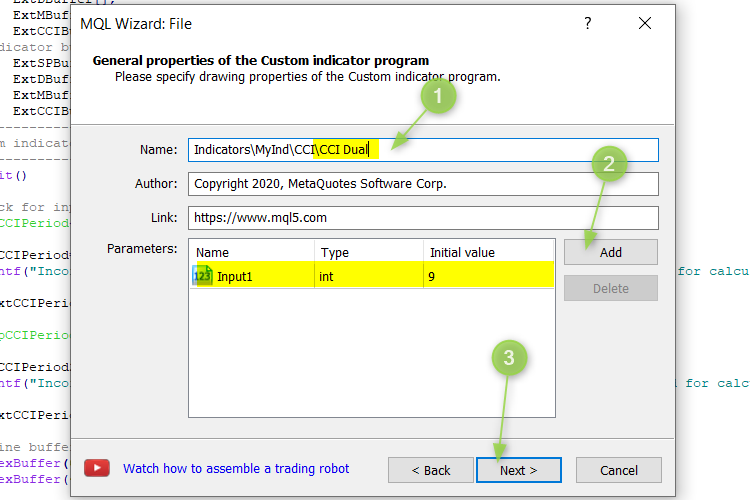Fig. 1. Step one

1. Give a name to the indicator
2. Add an input parameter (then replace it)
3. Click the ' Next ' button

Full text and code: CCI Dual:

### Simple example One position

Code: Simple example One position.mq5

```//+------------------------------------------------------------------+
//|                                  Simple example One position.mq5 |
//+------------------------------------------------------------------+
#property version   "1.000"
/*
*/
//---
CPositionInfo  m_position;                   // object of CPositionInfo class
CSymbolInfo    m_symbol;                     // object of CSymbolInfo class
//--- input parameters
input ENUM_POSITION_TYPE   InpPosType           = POSITION_TYPE_BUY; // Open position
input ulong                InpDeviation         = 10;                // Deviation
input ulong                InpMagic             = 200;               // Magic number
//+------------------------------------------------------------------+
//| Expert initialization function                                   |
//+------------------------------------------------------------------+
int OnInit()
{
//---
if(!m_symbol.Name(Symbol())) // sets symbol name
{
Print(__FILE__," ",__FUNCTION__,", ERROR: CSymbolInfo.Name");
return(INIT_FAILED);
}
//---
//---
return(INIT_SUCCEEDED);
}
//+------------------------------------------------------------------+
//| Expert deinitialization function                                 |
//+------------------------------------------------------------------+
void OnDeinit(const int reason)
{
//---
}
//+------------------------------------------------------------------+
//| Expert tick function                                             |
//+------------------------------------------------------------------+
void OnTick()
{
//---
if(!IsPositionExists())
{
else
}
}
//+------------------------------------------------------------------+
//| Is position exists                                               |
//+------------------------------------------------------------------+
bool IsPositionExists(void)
{
for(int i=PositionsTotal()-1; i>=0; i--)
if(m_position.SelectByIndex(i)) // selects the position by index for further access to its properties
if(m_position.Symbol()==m_symbol.Name() && m_position.Magic()==InpMagic)
return(true);
//---
return(false);
}
//+------------------------------------------------------------------+
```

The EA holds one position in the market (of a given type) - as soon as you close this position, the EA will open one position again.

Files:

### Example MultiTimeFrame iRSI get value

Code: Example MultiTimeFrame iRSI get value.mq5

It should be remembered that in MQL5 the indicator handle must be received once (in OnInit). Objective: there are three timeframes and their own parameters for RSI indicators. You need to get values from these indicators.

Algorithm of actions: at the global program level (in the "header" of the Expert Advisor), declare the 'arr_handle_iRSI' array - this array will store the handles of RSI indicators from different time frames.

Code:

```//+------------------------------------------------------------------+
//|                        Example MultiTimeFrame iRSI get value.mq5 |
//+------------------------------------------------------------------+
#property version   "1.000"
/*
*/
//--- input parameters
input group             "RSI 0"
input bool                 Inp_RSI_0_Use           = true;        // RSI 0: Use
input ENUM_TIMEFRAMES      Inp_RSI_0_period        = PERIOD_M5;   // RSI 0: timeframe
input int                  Inp_RSI_0_ma_period     = 14;          // RSI 0: averaging period
input ENUM_APPLIED_PRICE   Inp_RSI_0_applied_price = PRICE_CLOSE; // RSI 0: type of price
input group             "RSI 1"
input bool                 Inp_RSI_1_Use           = true;        // RSI 1: Use
input ENUM_TIMEFRAMES      Inp_RSI_1_period        = PERIOD_H1;   // RSI 1: timeframe
input int                  Inp_RSI_1_ma_period     = 14;          // RSI 1: averaging period
input ENUM_APPLIED_PRICE   Inp_RSI_1_applied_price = PRICE_CLOSE; // RSI 1: type of price
input group             "RSI 2"
input bool                 Inp_RSI_2_Use           = true;        // RSI 2: Use
input ENUM_TIMEFRAMES      Inp_RSI_2_period        = PERIOD_D1;   // RSI 2: timeframe
input int                  Inp_RSI_2_ma_period     = 14;          // RSI 2: averaging period
input ENUM_APPLIED_PRICE   Inp_RSI_2_applied_price = PRICE_CLOSE; // RSI 2: type of price
input bool     InpPrintLog          = false;       // Print log
//---
int               arr_handle_iRSI[];                     // array for storing the handle of the iRSI indicator
ENUM_TIMEFRAMES   arr_timeframe_iRSI[];                  // array for storing the timeframe of the iRSI indicator
//+------------------------------------------------------------------+
//| Expert initialization function                                   |
//+------------------------------------------------------------------+
int OnInit()
{
//---
if(Inp_RSI_0_Use)
{
//--- create handle of the indicator iRSI
int handle_iRSI=iRSI(Symbol(),Inp_RSI_0_period,Inp_RSI_0_ma_period,Inp_RSI_0_applied_price);
//--- if the handle is not created
if(handle_iRSI==INVALID_HANDLE)
{
//--- tell about the failure and output the error code
PrintFormat("Failed to create handle of the iRSI indicator for the symbol %s/%s, error code %d",
Symbol(),
EnumToString(Inp_RSI_0_period),
GetLastError());
//--- the indicator is stopped early
return(INIT_FAILED);
}
int size=ArraySize(arr_handle_iRSI);
ArrayResize(arr_handle_iRSI,size+1,3);
ArrayResize(arr_timeframe_iRSI,size+1,3);
arr_handle_iRSI[size]=handle_iRSI;
arr_timeframe_iRSI[size]=Inp_RSI_0_period;
}
//---
if(Inp_RSI_1_Use)
{
//--- create handle of the indicator iRSI
int handle_iRSI=iRSI(Symbol(),Inp_RSI_1_period,Inp_RSI_1_ma_period,Inp_RSI_1_applied_price);
//--- if the handle is not created
if(handle_iRSI==INVALID_HANDLE)
{
//--- tell about the failure and output the error code
PrintFormat("Failed to create handle of the iRSI indicator for the symbol %s/%s, error code %d",
Symbol(),
EnumToString(Inp_RSI_1_period),
GetLastError());
//--- the indicator is stopped early
return(INIT_FAILED);
}
int size=ArraySize(arr_handle_iRSI);
ArrayResize(arr_handle_iRSI,size+1,3);
ArrayResize(arr_timeframe_iRSI,size+1,3);
arr_handle_iRSI[size]=handle_iRSI;
arr_timeframe_iRSI[size]=Inp_RSI_1_period;
}
//---
if(Inp_RSI_2_Use)
{
//--- create handle of the indicator iRSI
int handle_iRSI=iRSI(Symbol(),Inp_RSI_2_period,Inp_RSI_2_ma_period,Inp_RSI_2_applied_price);
//--- if the handle is not created
if(handle_iRSI==INVALID_HANDLE)
{
//--- tell about the failure and output the error code
PrintFormat("Failed to create handle of the iRSI indicator for the symbol %s/%s, error code %d",
Symbol(),
EnumToString(Inp_RSI_2_period),
GetLastError());
//--- the indicator is stopped early
return(INIT_FAILED);
}
int size=ArraySize(arr_handle_iRSI);
ArrayResize(arr_handle_iRSI,size+1,3);
ArrayResize(arr_timeframe_iRSI,size+1,3);
arr_handle_iRSI[size]=handle_iRSI;
arr_timeframe_iRSI[size]=Inp_RSI_2_period;
}
//---
return(INIT_SUCCEEDED);
}
//+------------------------------------------------------------------+
//| Expert deinitialization function                                 |
//+------------------------------------------------------------------+
void OnDeinit(const int reason)
{
//---
}
//+------------------------------------------------------------------+
//| Expert tick function                                             |
//+------------------------------------------------------------------+
void OnTick()
{
//---
int size=ArraySize(arr_handle_iRSI);
if(size==0)
return;
//---
string text="";
for(int i=0; i<size; i++)
{
double rsi[];
ArraySetAsSeries(rsi,true);
int start_pos=0,count=3;
if(!iGetArray(arr_handle_iRSI[i],0,start_pos,count,rsi))
return;
//---
text=text+EnumToString(arr_timeframe_iRSI[i]);
int limit=(count>3)?3:count;
for(int j=0; j<limit; j++)
{
text=text+
" bar#"+IntegerToString(j)+": "+
" rsi "+DoubleToString(rsi[j],2);
}
text=text+"\n";
}
Comment(text);
}
//+------------------------------------------------------------------+
//| Get value of buffers                                             |
//+------------------------------------------------------------------+
bool iGetArray(const int handle,const int buffer,const int start_pos,
const int count,double &arr_buffer[])
{
bool result=true;
if(!ArrayIsDynamic(arr_buffer))
{
if(InpPrintLog)
PrintFormat("ERROR! EA: %s, FUNCTION: %s, this a no dynamic array!",__FILE__,__FUNCTION__);
return(false);
}
ArrayFree(arr_buffer);
//--- reset error code
ResetLastError();
//--- fill a part of the iBands array with values from the indicator buffer
int copied=CopyBuffer(handle,buffer,start_pos,count,arr_buffer);
if(copied!=count)
{
//--- if the copying fails, tell the error code
if(InpPrintLog)
PrintFormat("ERROR! EA: %s, FUNCTION: %s, amount to copy: %d, copied: %d, error code %d",
__FILE__,__FUNCTION__,count,copied,GetLastError());
//--- quit with zero result - it means that the indicator is considered as not calculated
return(false);
}
return(result);
}
//+------------------------------------------------------------------+
```

Result of work: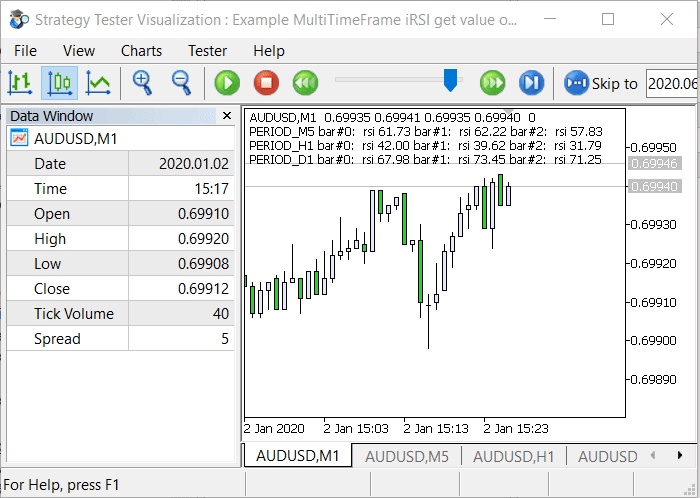Files: